The Vietnamese Mathematical Hobby Group

MATHEMATICAL TEST PAPER #1

Question 1

(i) Find the second derivative of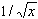(ii) Find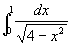(iii) Write down the equation satisfies by all points in the x-y plane at a distance of two units from the point (3, 4).

(iv) The curve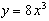is reflected in the line y = x . Find the equation of the resulting curve.

Question 2:

(i) Find the area under the curve y = 1/x between x = e and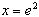.

(ii) It is known that the equation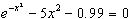has a positive root close to the origin. Attempt to find the root using Newton's method starting with initial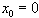. Explain why this method fails.

(iii) Find the derivative of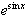.

Question 3:

(i) A die has the numbers 1, 2, 3, 4, 5 and 6 marked on its 6 faces. Find the expected value of the number which is obtained by casting the die at random.

(ii) Does the curve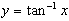has a maximum ? Explain your answer with the aid of a sketch.

(iii) Write down the sum S of the geometric series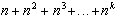where k is a positive integer. Does S approach a limit as n tends to infinity, k remaining constant.

Question 4:

Consider the parabola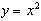(i) Find the equation of the tangent l to the parabola at the point P(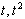).

(ii) Show that the line passing through the focus of the parabola and perpendicular to l has the equation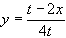(iii) Hence, or otherwise, find the locus of the foot of the perpendicular from the focus to the tangent to the parabola at any point.

Question 5:

A function f is called odd if f(-x) = -f(x) for all x.

(i) Prove that every odd function is zero at x=0.

(ii) Prove that every odd polynomial P(x) is divisible by x.

(iii) The polynomial P(x) is known to be monic, to be an odd function, and to have a root at x=5. Show that P(x) has degree no less than 3.

(iv) Find a polynomial Q(x) of degree 3 with the properties given in part (iii). State, with reason, whether there are any other polynomials of degree 3 with these properties.

(v) State the form of the most general polynomial with the properties given in part (iii) and with degree d in the range 4 < d < 6.

Question 6:

A particle moving along the x-axis starts at the origin with an initial velocity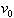. Its acceleration is given by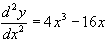(i) Show that the quantity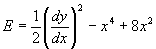does not change with time.

(ii) Given that the initial velocity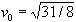, find the value of E.

(iii) Hence, or otherwise, establish that the particle with this initial velocity remains at all times in the region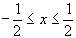. State, giving your reason, whether the motion is simple harmonic.

(iv) Find the value of E when the initial velocity is= 6. Use this result to discuss the nature of the subsequent motion.

Question 7:

A deck of cards consist of 4 groups of 7 cards, each group having a distinctive colour. The cards within any one group are labelled with the integers "1" through "7".

(i) Determine the total number of different five-card subsets that can be chosen from this deck. Leave your answer unsimplified.

(ii) Find the probability that five cards selected at random consist of exactly four cards labelled with the same integer, plus one other card. Express your answer as un unsimplified fraction.

(iii) The five cards are replaced in the deck, and the deck is shuffled. Five cards are selected at random. Find the probability that these five cards consist of three cards of which is labelled with the integer "2", plus two cards each of which is labelled with the integer "6". Express your answer as an unsimplified fraction.

Question 8:

(i) Write down an expression for cos(a + b) and hence prove that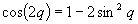.

(ii) Prove the identity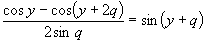(iii) Use math. induction and the result of (ii) to prove that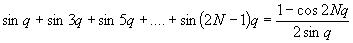.

Question 9:

Consider the integral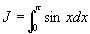(i) Determine the value of J.

(ii) By dividing the interval from x = 0 to x =p into N equal subintervals and using the midordinate approximation, show that an approximate value for J is given by: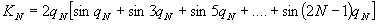where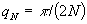.

(iii) Evaluate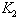to two significant figures; compare this with the exact value of J and state the percentage error of the approximation, to the nearest percent.

(iv) Starting with the formula of part (ii) and using the result stated in question 8(iii) deduce the exact value of J. (It may be assumed that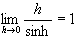).